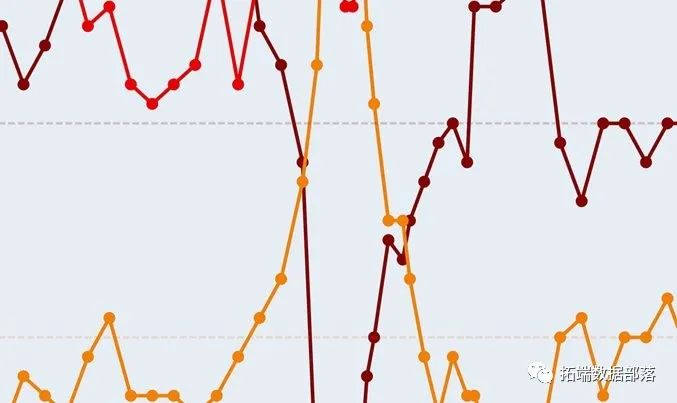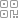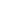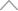5 9 0 2 9 4 5

# 设置工作目录

setwd("/Users/")

# 加载所需的软件包。

library(ggplot2) # 用于绘制一些初始图。

library(forecast) # 用于时间序列的预测。

# 创建一个在谷歌分析查询中使用的参数列表

listparam = Int(

dinsns= "ga:date",

mercs = "ga:users",

sot = "ga:date",

maresults = 10000,

tae.id = "ga:99395442"

)

# 存储谷歌分析的查询结果

es = QueyBlder(lit_pram)

df = GetRporData(rs, oaut_tken, splidawse = T)# 对结果重新排序

# 检查前30天的用户# 处理日期和绘制每日用户

df\$dte <- as.ate(dfdte, '%m%d')

df\$d = as. fator(weekays(dfdate))

ggplot( daa = df, as( dateusers )) +

geom_line()01020304# 创建工作日作为因素并绘制它

df\$wd = fator(df\$kd)

ggplot(df, aes(x=wd, y=srs)) +

geom_boxplt()# 将数据框转换成时间序列对象

dfts = ts(df\$ers, freqny = 7)

# 分解时间序列并绘制结果

dcmp = dompose(dfts, tye = "aditve")

prit(dcmp)plot(decp)# 在时间序列中应用HoltWinters模型并检查拟合情况

print(pred)# 绘制模型拟合图

plot(pred)#对未来用户的预测

forecast(pred, h=28)# 模型残差的相关图和柱状图

acf(residuals[8:length])## 新知精选

IT研发知识▪优秀创作者

### 优选课程 新知学院

•扫码下载商业新知APP~

•回到顶部